Copyright © Michael Richmond. This work is licensed under a Creative Commons License.

# Celestial Coordinates

Right Ascension (or "RA") and Declination (or "Dec") are global coordinates: any particular star has the same RA and Dec for all observers on Earth, and that position remains the same, night after night. Altitude and Azimuth, on the other hand, are local coordinates: each observer sets up his own reference frame. Moreover, the altitude and azimuth of a given star will change over just a few minutes as the star appears to rise, move across the sky, and set.

#### Right Ascension and Declination

On Earth, one way to describe a location is with a coordinate system which is fixed to the Earth's surface.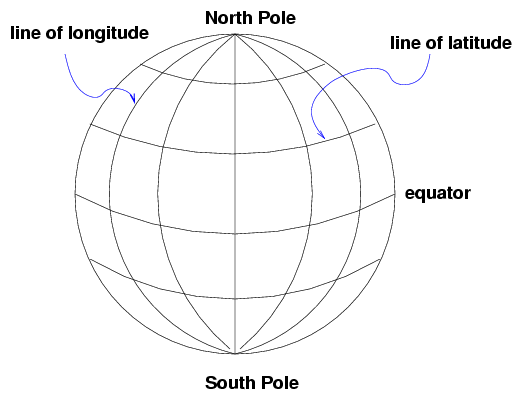The system is oriented by the spin axis of the Earth, and has special points at the North and South Poles. We use lines of latitude and longitude to demarcate the surface. It's obvious that latitude is measured away from the equator. But where is the starting point for longitude? There is no "obvious" choice. After a lot of dickering, European nations finally decided to use the location of the Greenwich Observatory in England as the starting point for longitude.

There are several ways to specify a location -- for example, that of the RIT Observatory. One can use degrees:

```
latitude 43.0758 degrees North, longitude 77.6647 degrees West of Greenwich
```

Or degrees, minutes and seconds:

```
latitude 43:04:33 North, longitude 77:39:53 West
```

Or, in the case of longitude, one can measure in time zones. The sun will set at the RIT Observatory about 5 hours and 11 minutes later than it does at Greenwich, so one could say

```
latitude 43:04:33 North, longitude 05 hours 11 minutes West
```

#### The celestial coordinates

On can make a similar coordinate system which is "fixed to the sky":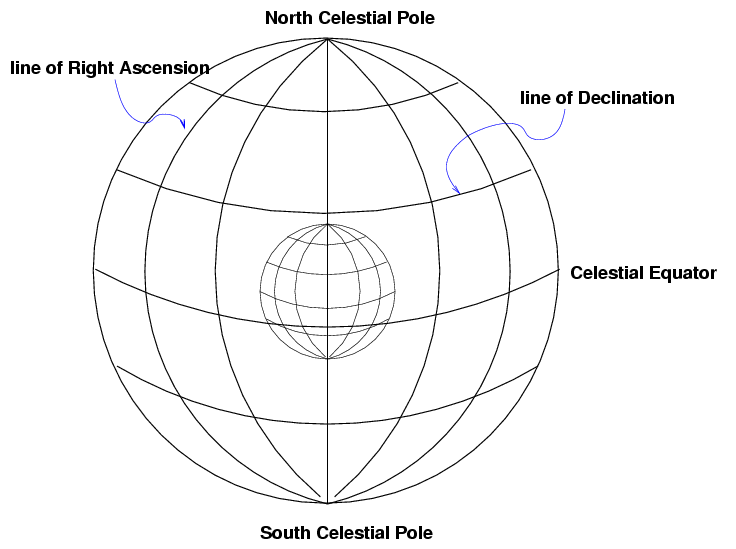Once again, we use the Earth's rotation axis to orient the coordinates. There are two special places, the North and South Celestial Poles. As the Earth rotates (to the East), the celestial sphere appears to rotate (to the West). Stars appear to move in circles: small ones near the celestial poles, and large ones close to the celestial equator:

We again use two orthogonal coordinates to describe a position:

• Declination, like a celestial latitude
• Right Ascension, like a celestial longitude
As with latitude, Declination is measured away from the celestial equator. But there is again no obvious choice for the starting point of the other set of coordinates. Where should we start counting Right Ascension? The rather arbitrary choice made by astronomers long ago was to pick the point at which the Sun appears to cross the celestial equator from South to North as it moves through the sky during the course of a year. We call that point the "vernal equinox".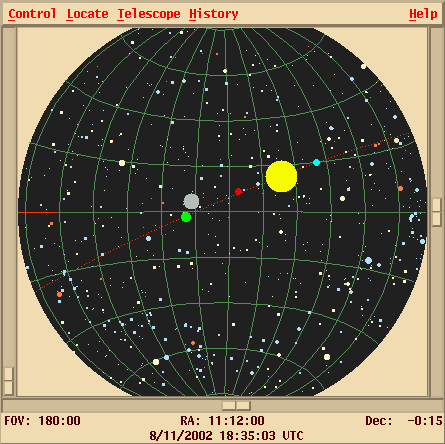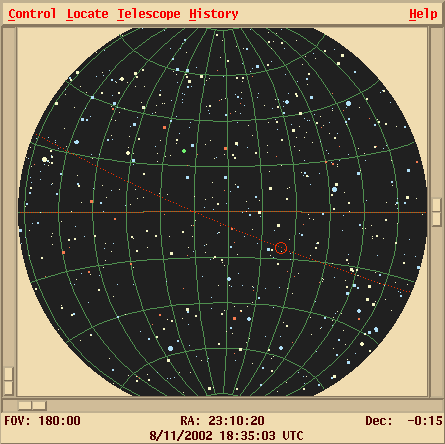Once again, there are several ways to express a location. The star Sirius, for example, can be described as at

```
Right Ascension 101.287 degrees, Declination -16.716 degrees
```

We can also express the Declination in Degrees:ArcMinutes:ArcSeconds, just as we do for latitude; and, as usual, there are 360 degrees around a full circle. For Right Ascension, astronomers always use the convention of Hours:Minutes:Seconds. There are 24 hours of RA around a circle in the sky, because it takes 24 hours for the Sun to move all the way from sunrise to the next sunrise.

```
Right Ascension 06:45:09, Declination -16:42:58
```
meaning
• the Right Ascension is 6 hours, 45 minutes, 09 seconds
• the Declination is -16 degrees, 42 arcminutes, 58 arcseconds

What's the difference between an "arcminute" and a "minute"? Units with the prefix "arc" refer to geometric angles:

• a circle is divided into 360 degrees
• one degree is divided into 60 arcminutes
• one arcminutes is divided into 60 arcseconds. Therefore, there are 3600 arcseconds in one degree

On the other hand, a "minute" without the "arc" refers to the distance moved by an object due to the Earth's rotation. The Earth takes (roughly) 24 hours to rotate, so

• 24 hours of time make a complete circle
• one hour of time is divided into 60 minutes of time
• one minute of time is divided into 60 seconds of time. Therefore, there are 3600 seconds of time in one hour of time

On the celestial equator, one hour of time corresponds to 15 degrees. However, far from the celestial equator, the distance an object moves in one hour can be much smaller.

#### Altitude and Azimuth

These two coordinates, altitude (or "alt") and azimuth (or "az"), are centered on the observer.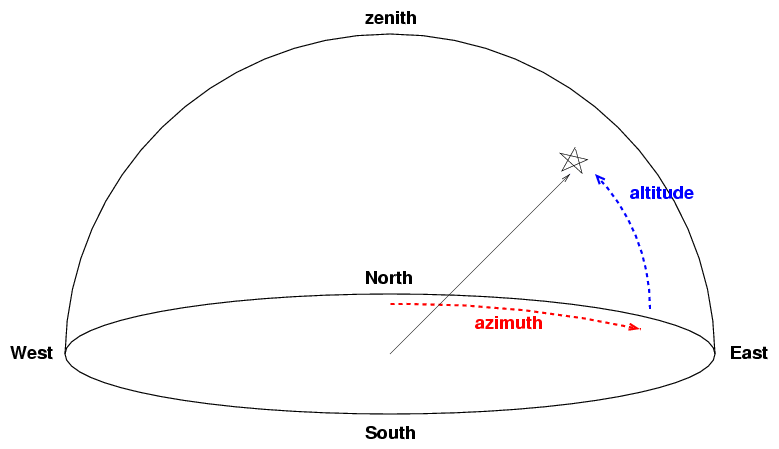• Altitude is the angular distance of an object above the local horizon. It ranges from 0 degrees at the horizon to 90 degrees at the zenith, the spot directly overhead.
• Azimuth is the angular distance of an object from the local North, measured along the horizon. An object which is due North has azimuth = 0 degrees; due East is azimuth = 90 degrees; due South is azimuth = 180 degrees; due West is azimuth = 270 degrees.

These two angles specify uniquely the direction of any object in the sky. Some telescopes have alt-az mounts which swivel in these two perpendicular axes; camera tripods and tank turrets are other examples of alt-az devices.

The altitude of an object is especially important from an practical point of view: any object which has an altitude less than zero is below the horizon, and hence inaccessible. Moreover, the altitude of an object is related to its airmass, a measure of how much air the light from that object must traverse to reach the observer. The larger the airmass, the more light is scattered or absorbed by the atmosphere, and hence the fainter an object will appear. We'll deal with airmass at greater length a bit later.

However, note that two observers at different locations on Earth will not agree on the (alt, az) position of an object. Moreover, as the Earth rotates, an object in the sky appears to move from East to West, so its (alt, az) position changes from moment to moment.

It is possible to convert from (RA, Dec) to (alt, az), or vice versa. One needs to know two factors:

• the location of the observer on Earth
• the time of the observation
The calculations involve some spherical trigonometry. One can find the details in any good book on celestial mathematics, such as
• Textbook on Spherical Astronomy by W. M. Smart
• Computational Spherical Astronomy by L. G. Taff
• Spherical Astronomy by R. M. Green
• Practical Astronomy with your Calculator by P. J. Duffet-Smith
• Astronomical Formulae for Calculators by J. Meeus

In these modern times, it's usually easiest to use one of the many fine planetarium programs on a computer to do this work.

#### A very little spherical trigonometry

You've learned trigonometry in high school: sines, cosines, the Pythagorean Theorem, and all that jazz. However, unless you went to a really good high school, you probably restricted your calculations to planar geometry. Unfortunately, the sky is not a plane. We measure positions and coordinates on the inner surface of an imaginary sphere. That means that the old rules don't always work anymore. The subject of spherical trigonometry is not a simple one, but, in this course, we will only peek into it.

Given two vectors, a and b, what is the distance between them? On a plane, we can break up each vector into its components and use the Pythagorean theorem: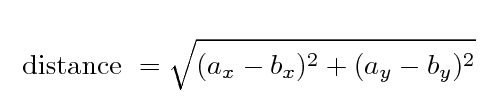Along the surface of the celestial sphere, if we want to find the angular separation between two points p1 and p2, we need to use the law of cosines. In the usual case, the two points are expressed in Right Ascension (α) and Declination (δ), like so: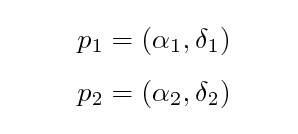In this case, the law of cosines becomes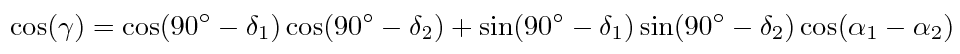which gives us the cosine of the desired angular distance γ.

If we are interested in very small angular distances on the sky -- the separation between the two components of a binary star, for example, or the distance between two of the moons of Jupiter -- then there are two common approximations. First, if we start with the RA and Dec coordinates of the two points, we can make a pseudo-Pythagorean formula; all we have to do is correct the difference in Right Ascension with the cosine of the Declination.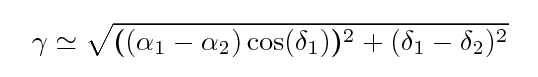Second, if we start with a picture of some very small region of the sky, together with an indication of the scale in arcseconds, like this: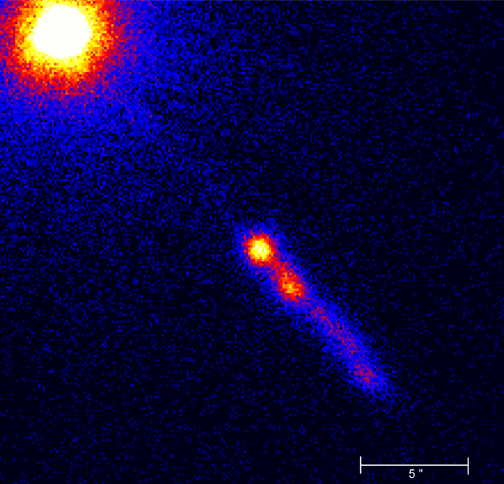then we can

• pick any two orthogonal directions on the picture
• measure the separation (in arcseconds) in each direction
• use the good old Pythagorean formula

#### Exercises

1. Polaris, the North Star, is close to Declination = +90 degrees. If you were standing on the Earth's North Pole, where would you see it in the sky?
2. If you were standing on the Equator, where would you see Polaris in the Sky?
3. The latitude of Rochester is +43 degrees North. How far above the horizon is Polaris as seen in Rochester?
4. What is the Declination of the most southern stars we can see in Rochester?
5. Convert the location of the bright star Regulus to decimal degrees:
```
RA  = 10:08:22.2    Dec = +11:58:02

Convert the coordinates of Arcturus to sexigesimal notation
(HH:MM:SS.s, DD:MM:SS.s)

RA  = 213.91317     Dec = +19.17897

How many degrees are there all the way around
the celestial equator?
How many arcseconds are there all the way around
the celestial equator?
What is the angular separation between these two locations, in degrees?

RA            Dec
-------------------------------
A      2 hours       0 degrees

B      5 hours       0 degrees
--------------------------------

What is the angular separation between these two locations, in degrees?

RA            Dec
-------------------------------
A      2 hours       50 degrees

B      5 hours       50 degrees
--------------------------------

(a tough one) What is the angular separation between the bright stars
Arcturus and Regulus?  Their coordinates are
provided in the questions above.
```Copyright © Michael Richmond. This work is licensed under a Creative Commons License.